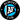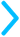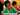## Inequality Graphs

Inequality graphs are just like regular graphs, except that you get to color.### Inequality Grapher

There are three easy steps to graphing an inequality:

1. Isolate y: It'll be easiest to graph our inequality if $y$ is on it's own on one side of the equals sign. Something like this:$y > 3x + 5$
And remember, if you have something like $-y < 3x + 5$, when you divide both sides by $-1$, you need to flip the sign, so that you end up with $y > 3x + 5$
Enter in an inequality to graph:
$y$